MATLAB Function Referencecontour

Contour graph of a matrix

Syntax

• ```contour(Z)
contour(Z,n)
contour(Z,v)
contour(X,Y,Z)
contour(X,Y,Z,n)
contour(X,Y,Z,v)
contour(...,`LineSpec`)
[C,h] = contour(...)

[C,h] = contour('v6',...)
```

Description

A contour graph displays isolines of matrix `Z`. Label the contour lines using `clabel`.

```contour(Z) ``` draws a contour plot of matrix `Z`, where `Z` is interpreted as heights with respect to the x-y plane. `Z` must be at least a 2-by-2 matrix. The number of contour levels and the values of the contour levels are chosen automatically based on the minimum and maximum values of `Z`. The ranges of the x- and y-axis are `[1:n]` and `[1:m]`, where `[m,n] = size(Z)`.

```contour(Z,n) ``` draws a contour plot of matrix `Z` with `n` contour levels.

```contour(Z,v) ``` draws a contour plot of matrix `Z` with contour lines at the data values specified in vector `v`. The number of contour levels is equal to `length(v)`. To draw a single contour of level `i`, use `contour(Z,[i i])`.

```contour(X,Y,Z), contour(X,Y,Z,n), and contour(X,Y,Z,v) ``` draw contour plots of `Z`. `X` and `Y` specify the x- and y-axis limits. When `X` and `Y` are matrices, they must be the same size as `Z`, in which case they specify a surface, as defined by the `surf` function.

If `X` or `Y` is irregularly spaced, `contour` calculates contours using a regularly spaced contour grid, then transforms the data to `X` or `Y`.

```contour(...,LineSpec) ``` draws the contours using the line type and color specified by `LineSpec`. `contour` ignores marker symbols.

```[C,h] = contour(...) ``` returns the contour matrix `C` (see `contourc`) and a handle to a contourgroup object. `clabel` uses the contour matrix `C` to create the labels. (See descriptions of contourgroup object properties.)

Backward Compatible Version

```[C,h] = contour('v6',...) ``` returns the contour matrix `C` (see `contourc`) and a vector of handles to graphics objects. `clabel` uses the contour matrix `C` to create the labels. `contour` creates patch graphics objects unless you specify a `LineSpec`, in which case `contour` creates line graphics objects.

See Plot Objects and Backward Compatibility for more information.

Remarks

If you do not specify the `LineSpec` argument, the figure colormap (`colormap`) and the color limits (`caxis`) control the color of the contour lines. In this case the `contour` function creates patch objects to implement the contour plot.

When you specify the `LineSpec` argument, the `contour` function creates line object to implement the contour plot. In this case, contour lines are not mapped to colors in the figure colormap, but are colored using the colors defined in the axes `ColorOrder` property.

Use contourgroup object properties to control the contour plot appearance.

The following diagram illustrates the parent-child relationship in contour plots.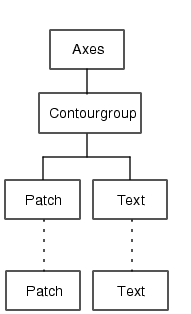Examples

Contour Plot of a Function

To view a contour plot of the function

•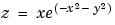over the range -2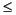x2, -2y3, create matrix `Z` using the statements

• ```[X,Y] = meshgrid(-2:.2:2,-2:.2:3);
Z = X.*exp(-X.^2-Y.^2);
```

Then, generate a contour plot of `Z`.

• Display contour labels by setting the `ShowText` property to `on`.
• Label every other contour line by setting the `TextStep` property to twice the contour interval (i.e., two times the `LevelStep` property).
• Use a smoothly varying colormap.
• ```[C,h] = contour(X,Y,Z);
set(h,'ShowText','on','TextStep',get(h,'LevelStep')*2)
colormap cool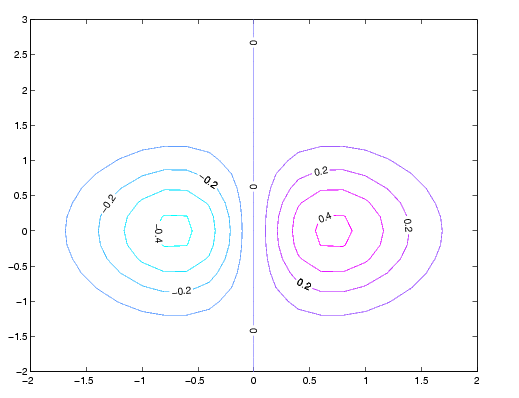```

Smoothing Contour Data

You can use `interp2` to create smoother contours. Also set the contour label text `BackgroundColor` to a light yellow and the `EdgeColor` to light gray.

• ```Z = peaks;
[C,h] = contour(interp2(Z,4));
text_handle = clabel(C,h);
set(text_handle,'BackgroundColor',[1 1 .6],...
'Edgecolor',[.7 .7 .7])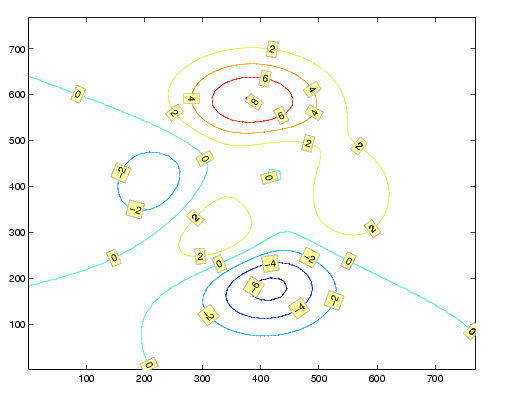```

Setting the Axis Limits on Contour Plots

Suppose, for example, your data represents a region that is 1000 meters in the x dimension and 3000 meters in the y dimension. You could use the following statements to set the axis limits correctly:

• ```Z = rand(24,36); ```% assume data is a 24-by-36 matrix
```X = linspace(0,1000,size(Z,2));
Y = linspace(0,3000,size(Z,1));
[c,h] = contour(X,Y,Z);
axis equal tight ```% set the axes aspect ratio
``````

See Also

`contour3`, `contourc`, `contourf`, `contourslice`

See Contourgroup Properties for poperty descriptions

© 1994-2005 The MathWorks, Inc.